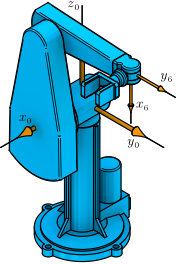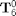# Extra Example:: Puma 560 Manipulator: Forward Kinematics (MATLAB)This example computes the forward kinematics for the Puma 560 manipulator. It uses the DH parameters reported by Paul and Zhang in Computationally Efficient Kinematics for Manipulators with Spherical Wrists Based on the Homogeneous Transformation Representation, 1986.

## Clear All Workspace Objects and Reset All Assumptions

clear all


## Joint Values

theta1 = 90; % deg
theta2 = 90; % deg
theta3 = -180; % deg
theta23 = theta2 + theta3; % deg
theta4 = 90; % deg
theta5 = 90; % deg
theta6 = -90; % deg


## Structural Parameters

a2 = 43.18; % cm
a3 = 1.91; % cm
d3 = 12.54; % cm
d4 = 43.18; % cm


## Local Transformation Variables

U512 = -cosd(theta5)*sind(theta6);
U522 = -sind(theta5)*sind(theta6);
U412 = -sind(theta4)*cosd(theta6) + cosd(theta4)*U512;
U413 = -cosd(theta4)*sind(theta5);
U422 = cosd(theta4)*cosd(theta6) + sind(theta4)*U512;
U423 = -sind(theta4)*sind(theta5);
U212 = cosd(theta23)*U412 - sind(theta23)*U522;
U213 = -sind(theta23)*cosd(theta5) + cosd(theta23)*U413;
U214 = a2*cosd(theta2) - d4*sind(theta23) + a3*cosd(theta23);
U222 = sind(theta23)*U412 + cosd(theta23)*U522;
U223 = cosd(theta23)*cosd(theta5) + sind(theta23)*U413;
U224 = a2*sind(theta2) + a3*sind(theta23) + d4*cosd(theta23);


## The 2nd Column ofU112 = U212*cosd(theta1) - U422*sind(theta1);
U122 = U212*sind(theta1) + U422*cosd(theta1);
j6Res0 = [U112; U122; U222];


## The 3rd Column ofU113 = U213*cosd(theta1) - U423*sind(theta1);
U123 = U213*sind(theta1) + U423*cosd(theta1);
k6Res0 = [U113; U123; U223];


## The 1st Column ofi6Res0 = cross(j6Res0, k6Res0);


## The 4th Column ofU114 = U214*cosd(theta1) + d3*sind(theta1);
U124 = U214*sind(theta1) - d3*cosd(theta1);
X6Rel0Res0 = [U114; U124; U224];


## The Forward Kinematics as the Homogeneous TransformationT60 = [i6Res0 j6Res0 k6Res0 X6Rel0Res0;
0      0      0      1]

T60 =

0         0    1.0000   12.5400
0    1.0000         0   43.1800
-1.0000         0         0   41.2700
0         0         0    1.0000



This MATLAB example illustrates a computation from the textbook Fundamentals of Robot Mechanics by G. L. Long, Quintus-Hyperion Press, 2015. See http://www.RobotMechanicsControl.info for additional relevant files.